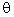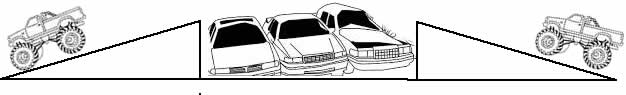#### Projectile Motion

Projectile motion is the motion of an object launched or propelled at an anglewith respect to the horizontal

The standard equations describing the motion of an object in two dimensions can be applied to this special case of accelerated motion which occurs,  in the vertical direction, under the influence of the acceleration due to gravity.

This is also a case of non-accelerated motion (only) in the horizontal direction.

The animation below describes the properties of a typical example of a projectile along its path of motion (known as the trajectory).

Important points to realize when analyzing projectile motion:
• The projectile angle is• The initial velocity V is tangent to the path of motion at an anglewith respect to the horizontal therefore, it has horizontal and vertical components.
• The horizontal component of the initial velocity is called V1x; where V1x = V1 (cos)
• The vertical component of the initial velocity is called V1y; where V1y = V1 (sin)
• The trajectory (the path of the projectile) is a parabola.  The parabola is symmetrical about a point half way between the origin and the total horizontal distance traveled by the projectile.
• At the half time point (t1/2), the projectile reaches its maximum height.
• The total horizontal distance (from the origin to where the projectile lands) is called the range (R).  This is the total distance traveled by the projectile (horizontally) in the x-direction
• When the projectile lands at time t (the total flight time), its vertical distance is zero (ground level)
• A projectile path (because it is a parabolic trajectory) will have two roots
• Projectiles can be analyzed as a function [y = f(t)] of time t, and as a function of horizontal distance x [y = (fx)]
##### Important Physics principles of projectiles:
• The horizontal velocity Vx, is always constant -- not affected by gravity (i.e. ax = 0)
• The vertical velocity Vy,  is affected by gravity -- it is positive but decreasing for the first part of the trajectory and negative but increasing for the second part of the trajectory. (i.e. ay = -9.8 m/s2).
• At the top of its flight (at t1/2), the slope of the tangent to this parabola is zero, therefore the vertical  velocity Vat that point is zero.
• A projectile  launched at angleand an object dropped at the same time from the same height, will reach the ground at the same time.  See illustration below.

##### Equations of motion relating to projectilesNote the hints below each equation.  These are given so that you can identify the correct conditions to use each equation in solving problems.

 Projectile Motion equations and Linear Motion Equations Linear Equations of Motion Projectile Motion Analysis Horizontal Vertical V2 = V1 + at The horizontal velocity of a projectile is always constant V2y = V1y+ 2gt V22  = V12 + 2 ad V2y2 = V1y2 + 2g yd = V1t + 1/2 at2d = Vavt (use in non-accelerated motion conditions only) The horizontal distance is calculated by: x = V1t The Range (R) is the maximum horizontal distance:  R = V12 (sin 2)/g  This is known as the Range Equation The Height (h) can be calculated by: y = Vy + 1/2g(t2) To find the maximum height substitute Vy = 0; at any other point uset to find the height of the projectile. This equation can also be rearranged to solve for time - use the quadratic formula to get the final answer. Vav = (V1 + V2) / 2 To find the total time use y = 0 Maximum Height can be also be found by usingt = t1/2 Use in free fall to calculate height:d(y)= V1t + 1/2 gt2 V1x = V1 (cos) This gives you the initial horizontal velocity V1y = V1 (sin) This gives you the initial vertical velocity This gives you the instantaneous velocity at any point along the trajectory of the projectile Use Pythagoras' Theorem to find the magnitude of Vtot.  Use trigonometry  to find its direction. Substitute the acceleration a with g (where g = - 9.8 m/s2) when dealing with free fall or projectiles.Try some of Galileo's famous experiments with falling objects.  http://www.pbs.org/wgbh/nova/physics/galileo-experiments.htmlNow try this problem:

In a monster truck show ( use this YouTube video for reference https://www.youtube.com/watch?v=uLbBx_25y3U ), a truck is set to “jump” over several cars lined up side-by-side by racing up a ramp inclined at 180  to the horizontal at a speed of 40 m/s.  A ramp is setup on the other side of the cars, also inclined at 180.  If each car is 2.5 m wide, how many cars can the truck realistically jump over?